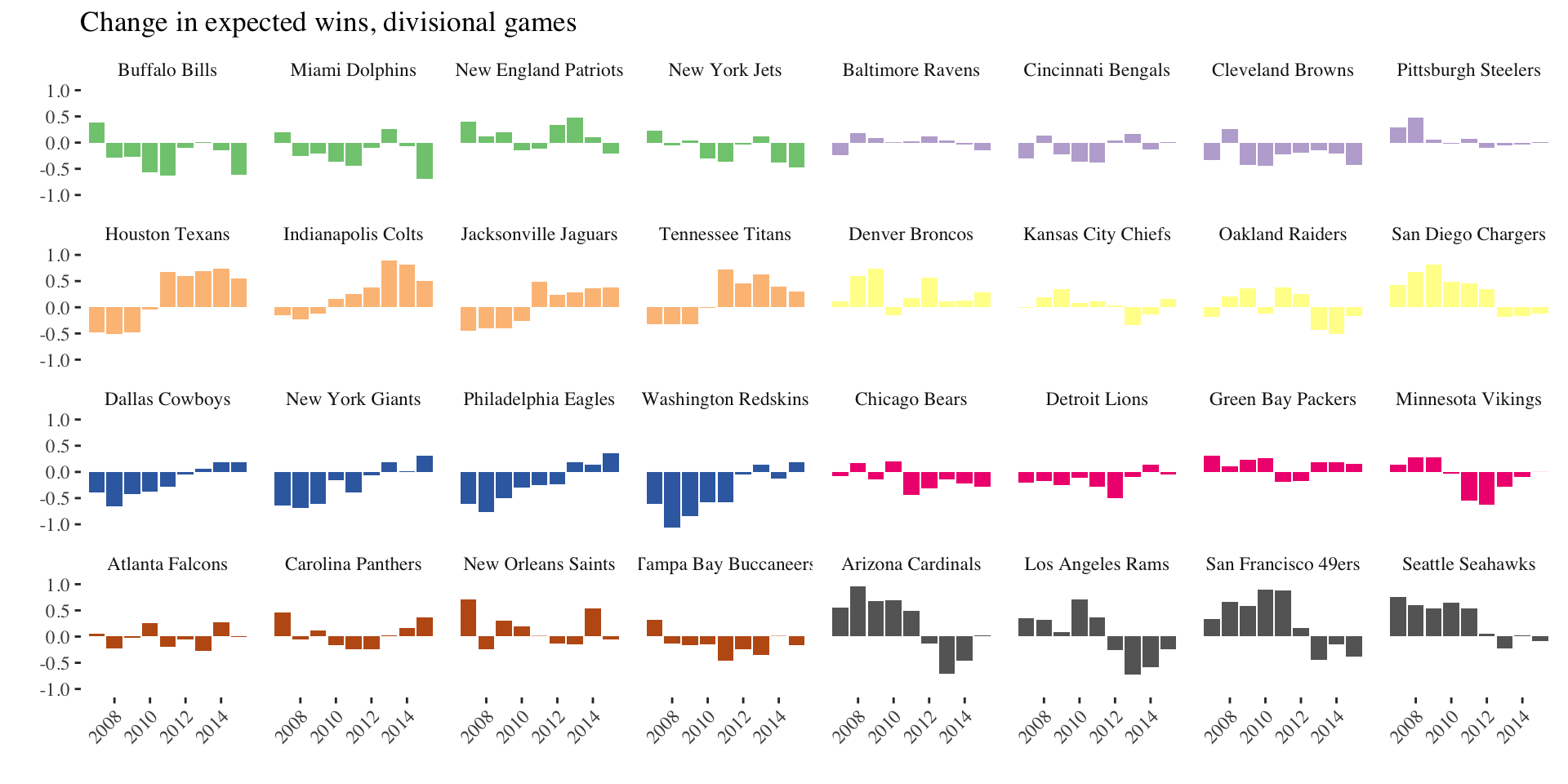Michael Lopez (with Gregory Matthews, Benjamin BaumerMichael Lopez, Gregory Matthews, Benjamin Baumer https://github.com/bigfour/competitiveness.

## Goals: the NFL’s

There are systems that we have to make sure that we maintain (parity).

Carefully constructed system of competitive balance that makes NFL games and championship races so unpredictable and exciting.

Roger Goodell, NFL commisioner

If you expect something to happen in this league, it’s likely not going to happen.

Jonathan Kraft, President of New England Patriots

## Goals: ours

1. Develop model to assess parity in professional sports
2. Does the NFL have parity?
3. How do NFL systems impact league outcomes?

## Assess parity in professional sports

How to define parity

the state or condition of being equal

## Assess parity in professional sports

What parity looks like: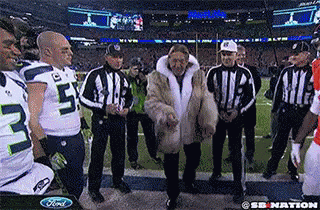## Assess parity in professional sports

What parity does not look like: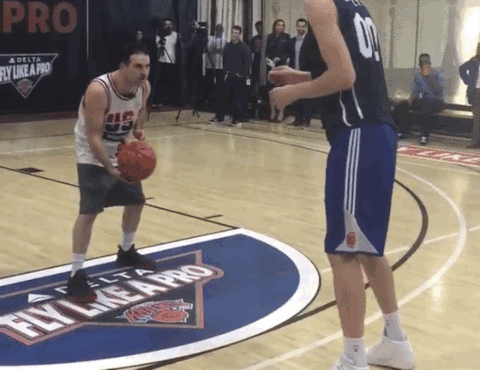## A cross-sport model to assess parity

Motivation: Extend Glickman and Stern, 1998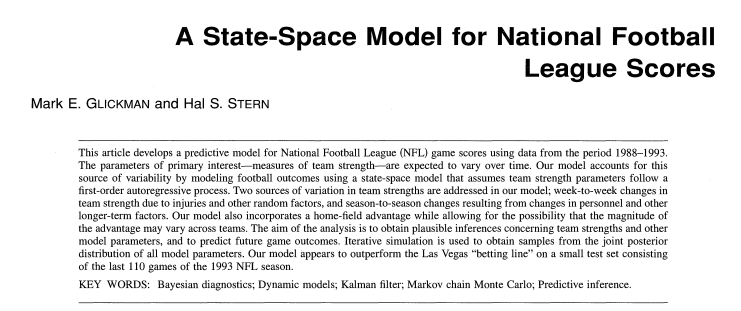## A cross-sport model to assess parity

• $$p_{(q,s,k)ij}$$ is probability that team $$i$$ will beat team $$j$$ in season $$s$$ during week $$k$$ of sports league $$q$$, for $$q \in \{MLB, NBA, NFL, NHL\}$$.
• $$\alpha_{q_{0}}$$ be the league-wide home advantage (HA) in $$q$$
• $$\alpha_{(q) i^{\star}}$$ be the extra effect (+ or -) for team $$i$$ when playing at home
• $$\theta_{(q,s,k) i}$$ and $$\theta_{(q, s, k) j}$$ be season-week team strength parameters

## A cross-sport model to assess parity

$E[\text{logit}(p_{(q,s,k) ij})] = \theta_{(q,s,k) i} - \theta_{(q, s, k) j} + \alpha_{q_0} + \alpha_{(q) i^{\star}}$

Assumptions:

1. $$\sum_{i=1}^{t_{q}} \theta_{(q,s,k)i} = 0$$
2. $$E[\theta_{(i,q,s+1,1)}] = \gamma_{q, season} \theta_{(i, q,s,k)}$$
3. $$E[\theta_{(i,q,s,k+1)}] = \gamma_{q, week} \theta_{(i, q,s,k)}$$
4. $$\gamma_{q,week}$$ and $$\gamma_{q,season}$$ week/season level autogressive parameters

## A cross-sport model to assess parity

What can our model tell us? A parity checklist

1. Unpredictability at a fixed time (variability of $$\theta_{(q,s,k) i}$$’s)
2. Within season unpredictability ($$\gamma_{q, week}$$)
3. Between season unpredictability ($$\gamma_{q, season}$$)

## A cross-sport model to assess parity

Data: 2006-2016 regular season games in MLB, NBA, NFL, NHL via Sports Insights

Priors: Uniform (variance parameters) and Normal (team strength parameters)

Software: rjags package in R statistical software

Draws: 20k iterations, 2k burn in, thin of 5

## Model estimates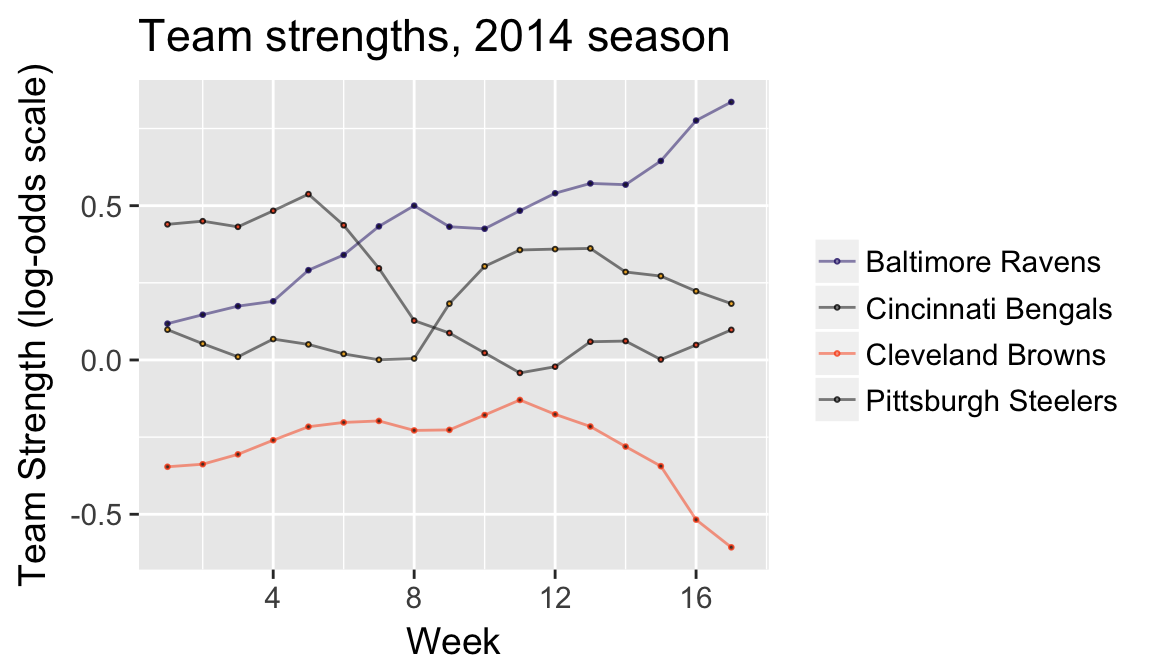## Model estimates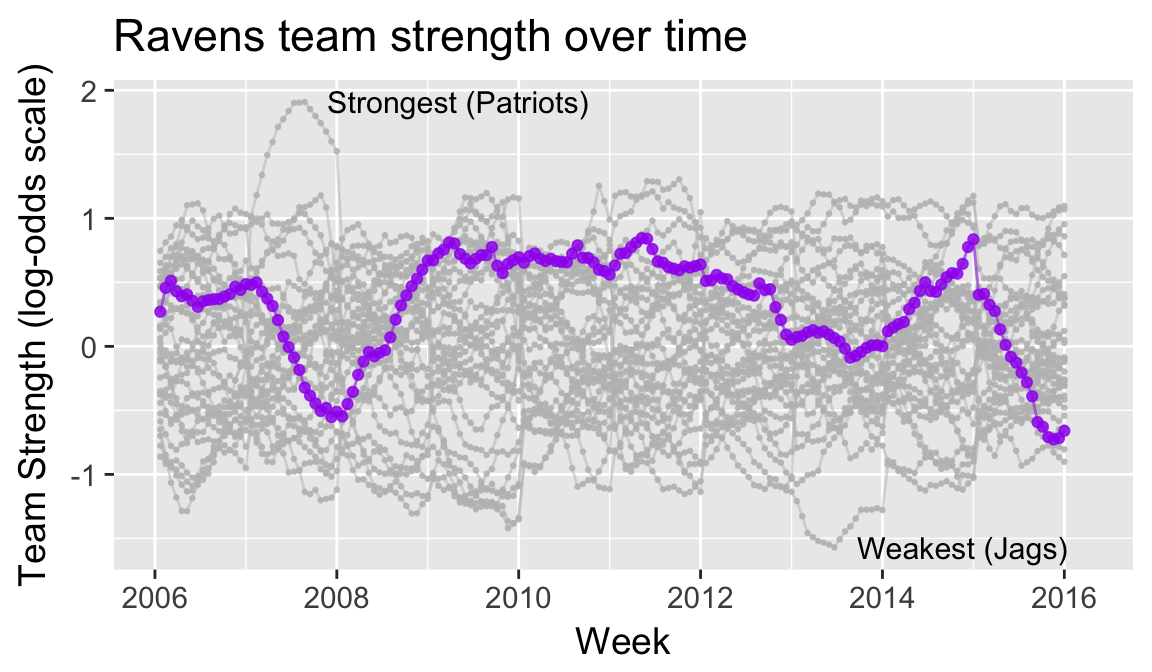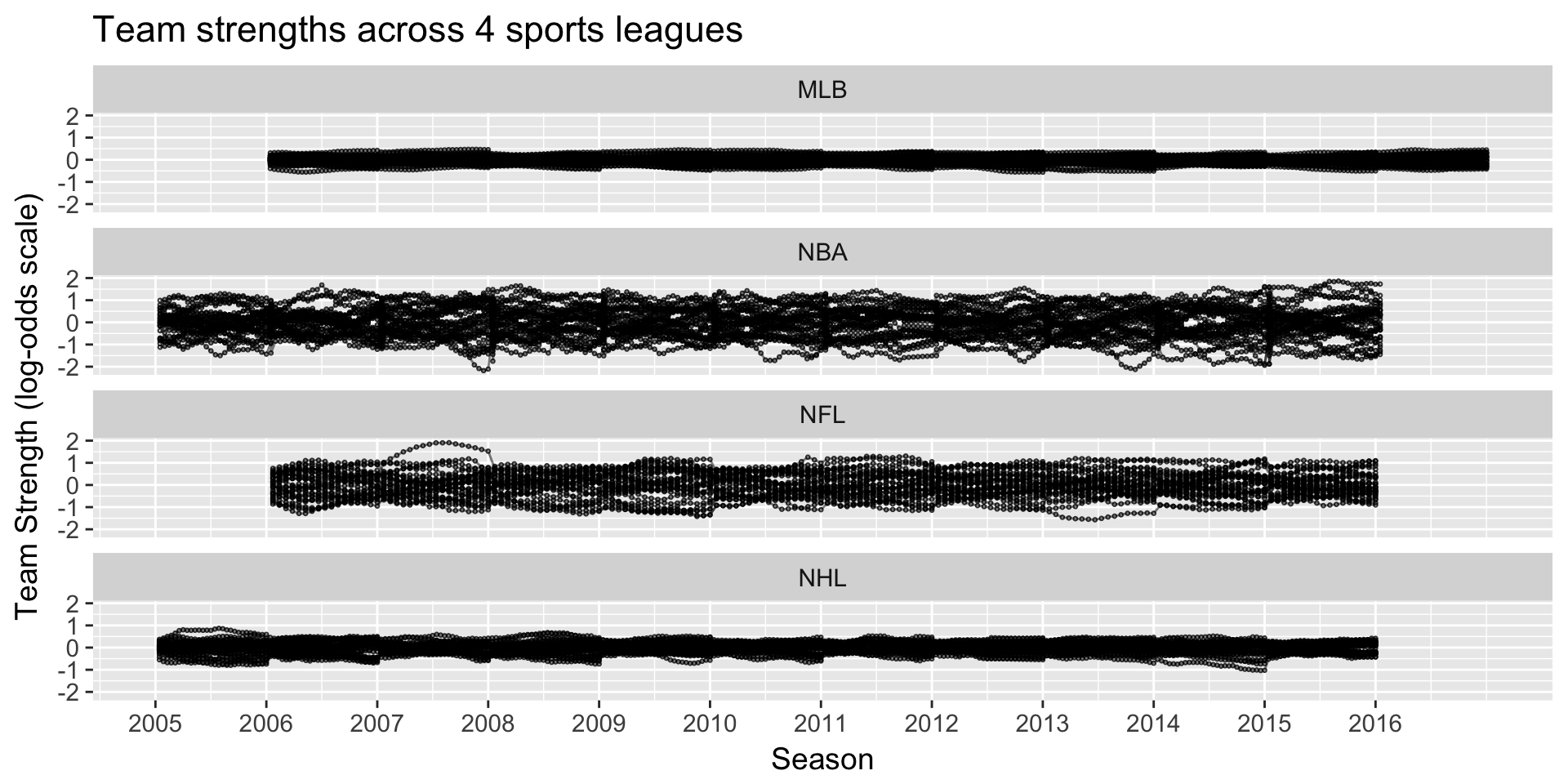## NFL’s parity: unpredictability at a fixed point in time

How often does the best team win?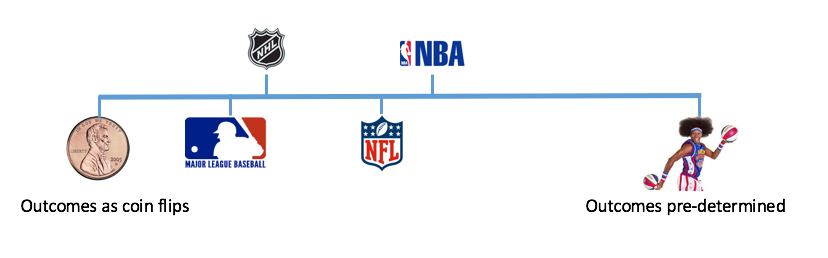## NFL’s parity: unpredictability by season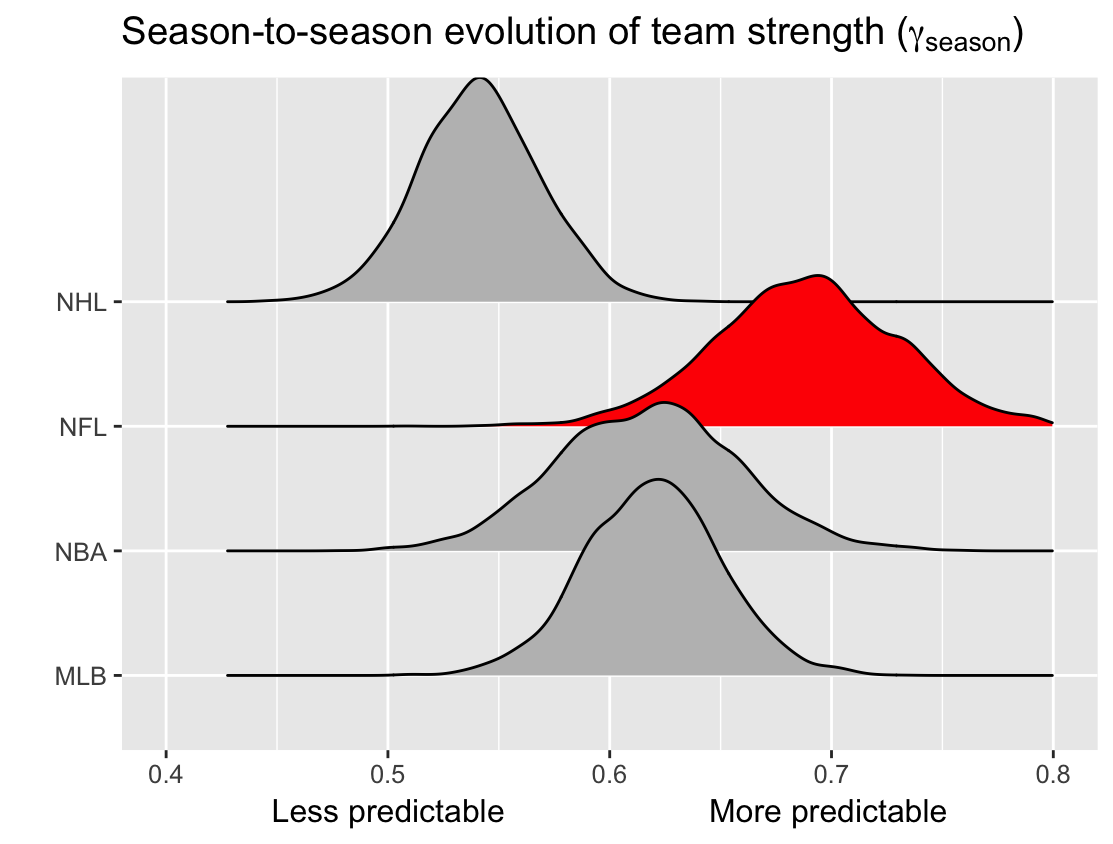## NFL’s parity: unpredictability by week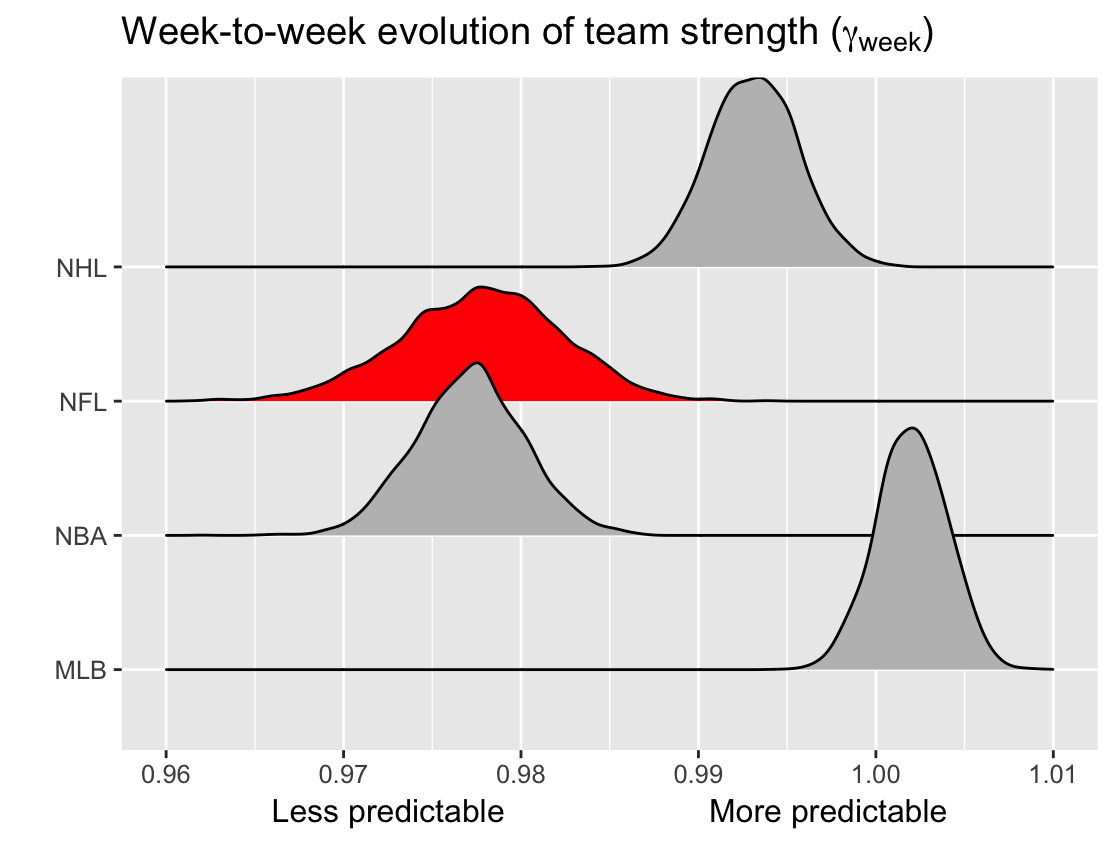## Effect of NFLs unbalanced schedule

2 games per year against like-placed teams from prior year (I’ll call them “crossover” games).

$$Hypothetical$$: What if league-average teams were played instead?

$$Approach$$: Use team strength estimates to approximate change in expected wins

## Effect of NFLs unbalanced schedule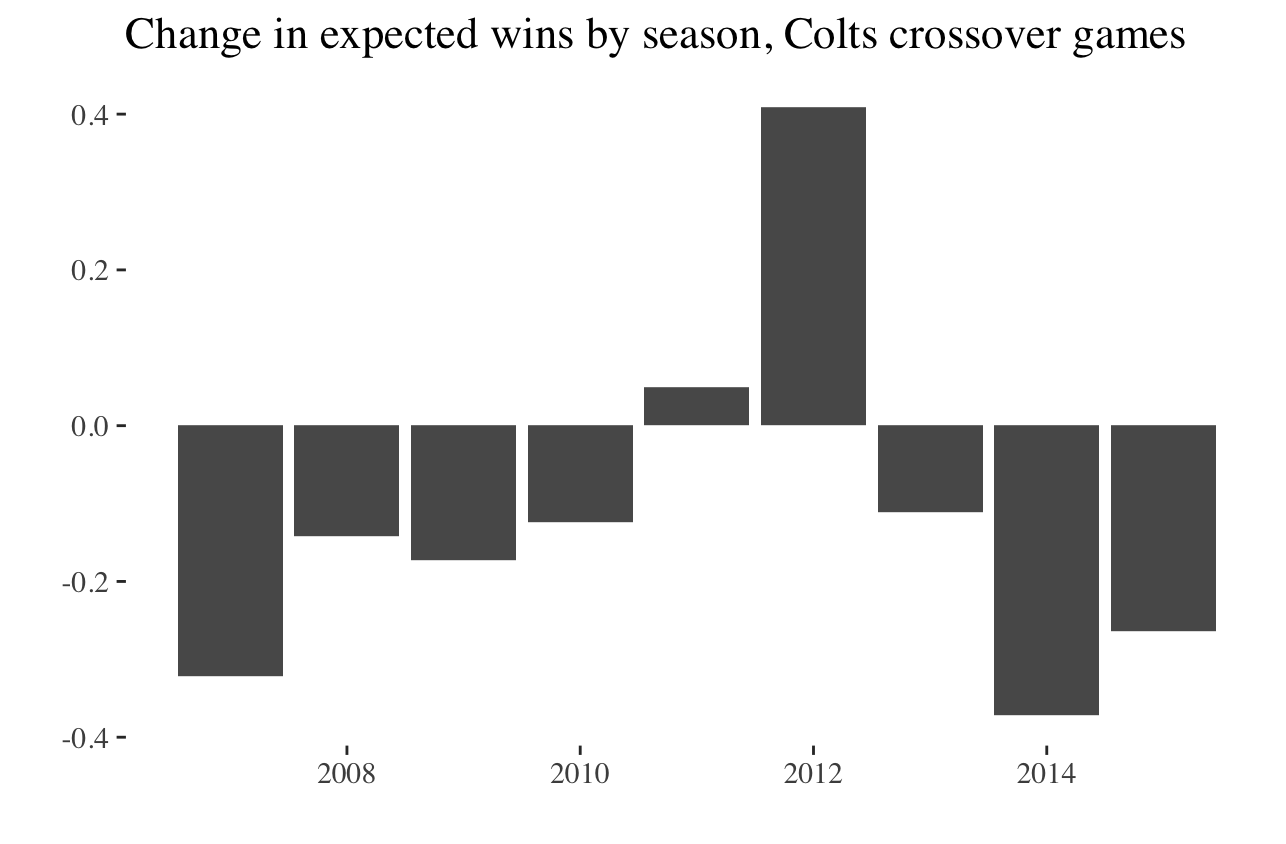## Effect of NFLs unbalanced schedule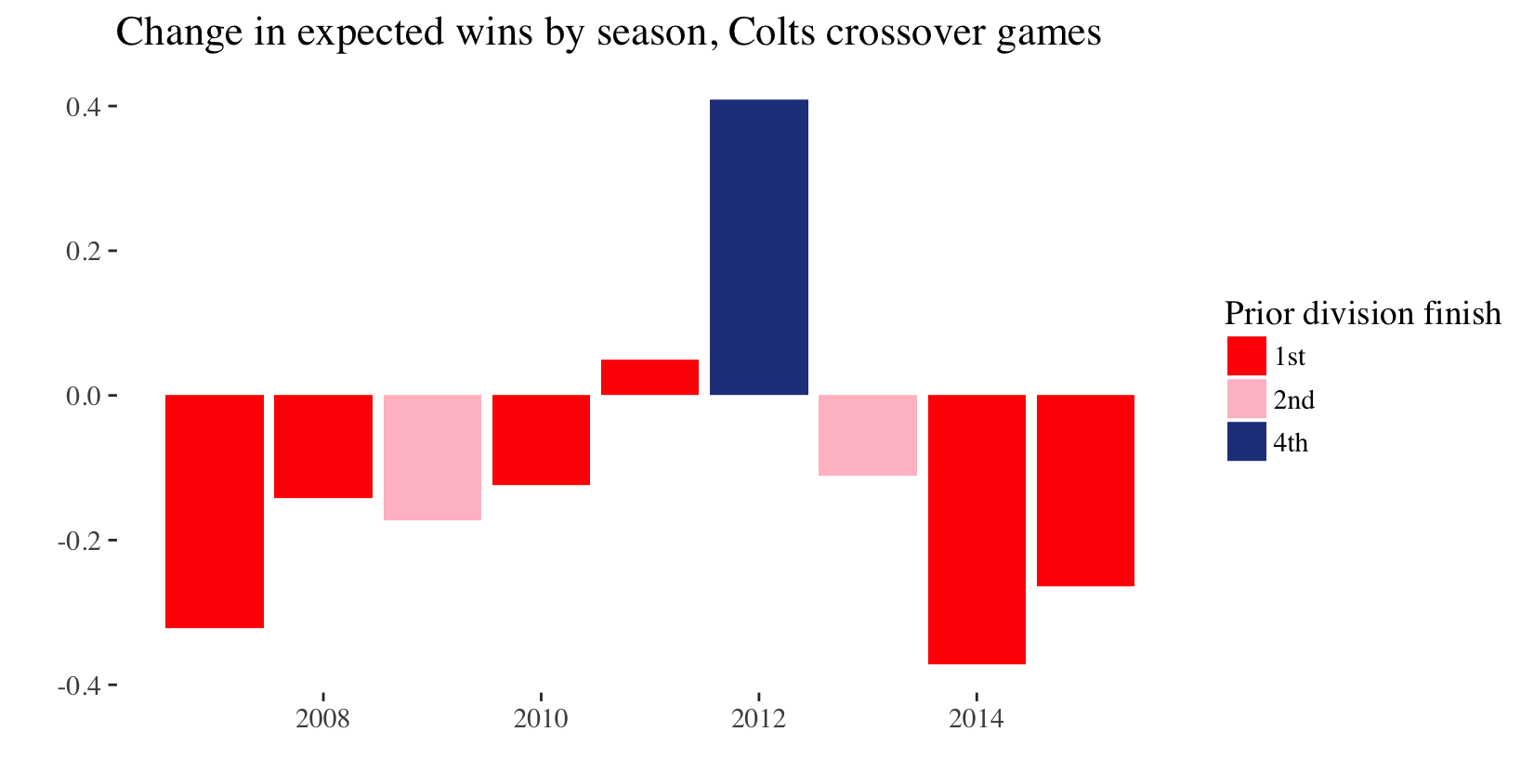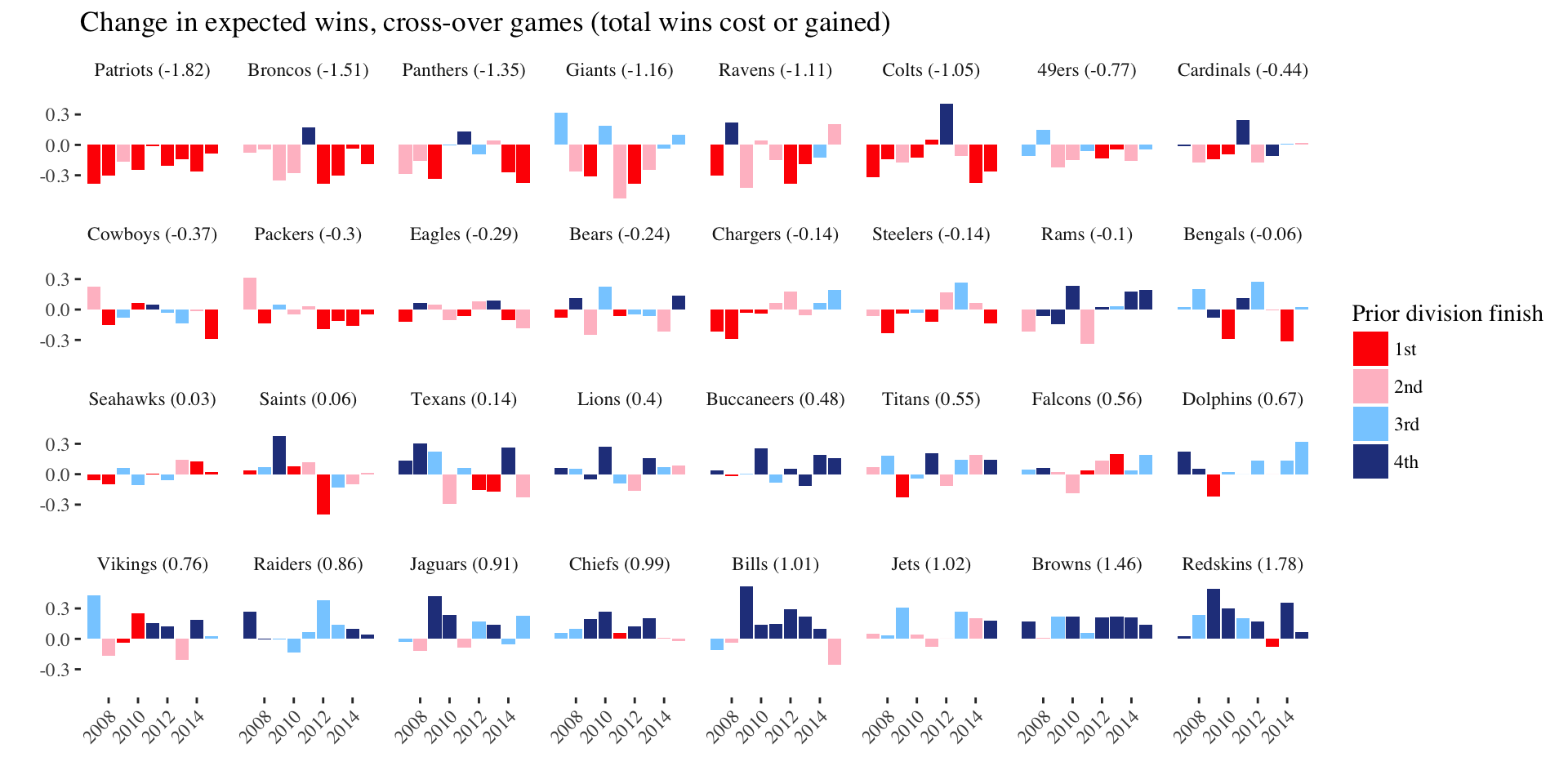## Conclusion

Does NFL have parity? Not particularly

## Conclusion

Do NFL systems push records to 0.500? Sort of

## Acknowledgements: Greg and Ben## Summary: parity in the NFL